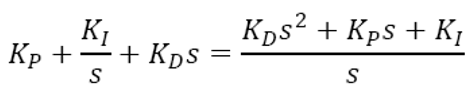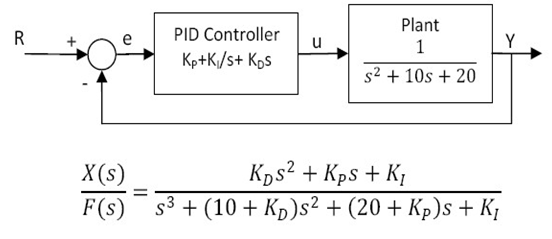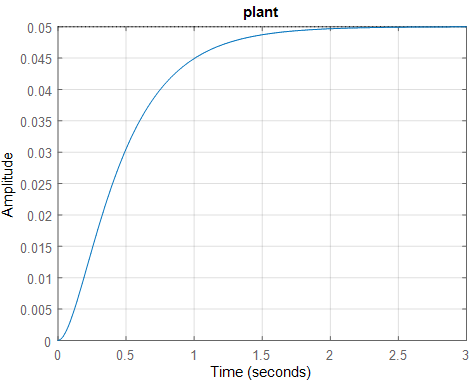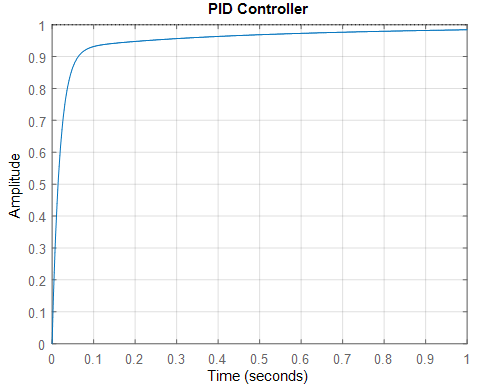Design PID controller Using MAtlab - Matlab Simulink for E & T

# PID Controller Using Matlab

Anyway going into subtleties, let us get a presentation about PID regulators by using matlab programming in editor window.

PID regulators are found in a wide scope of uses for mechanical cycle control.

Around 95% of the shut circle activities of modern robotization, industries, automation industries, electrical controlling etc as use PID regulators.

PID represents Proportional-Integral-Derivative. These three regulators are joined so that it creates a control signal.

For most suiatable laptop for Matlab-  https://amzn.to/39QeZ9d

Camera- https://amzn.to/39KBi02

Professional tripod- https://amzn.to/39Jg96w

## Plant:

A system to be controlled.

## Controller:

Provides excitation for the plant; Designed to control the overall system behavior.

## The transfer function of the PID controller looks like the following-KP = Proportional gain , KI = Integral gain ,  KD = Derivative gain

The closed loop transfer function of the given system with a PID controller is-

The gain Kp = 350 and Kd = 50 provided the desired response

Simple block diagram of PID controller and the simple equation of PID controller are## Matlab Code Program-

the design of PID controller using matlab follow some steps

>> open the editor window in matlab (shortcut key ctrl+N)

>> write down thw following matlab code in editor window

clc ,

clear ,

close all ;

num = 1 ;

den = [ 1 10 20] ;

plant = tf(num,den)

figure ,

step(plant)

grid ,

title('plant')

kp = 300 ;

% % PID controller

ki1 = 300 ;

kd1 = 50 ;

cont4 = tf([kd1 kp ki1],[1 0])

sys4 = feedback(cont4*plant,1)

figure ,

step(sys4,1)

grid ,

title(' PID Controller ')

after complete write code save the code and run for run press F5 key and output show in command window

## Output-

plant =

1

---------------

s^2 + 10 s + 20

Continuous-time transfer function.

cont4 =

50 s^2 + 300 s + 300

--------------------

s

Continuous-time transfer function.

sys4 =

50 s^2 + 300 s + 300

--------------------------

s^3 + 60 s^2 + 320 s + 300

Continuous-time transfer function.step response of plantstep response of PID controller

Related Post –

Design PIcontroller Using Matlab

For more matlab code and program

Please Like, Comment, follow our blog for new updates and Share and email subscribe for latest update

! THANK YOU !